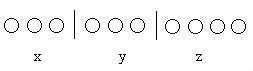2018-09-26 21:41:43（插板法）

$ans=a+b-c$

### 1.球相同，盒子不同，不能有空盒

$$ans=C^{m-1}_{n-1}$$

### 2.球相同，盒子不同，可以有空盒

$$ans=C^{m-1}_{n+m-1}$$

### 3.球不同，盒子不同，可以有空盒

$$ans=m^n$$

### 4.球不同，盒子相同，不能有空盒

$S2[i][j]=S2[i-1][j]*j+S2[i-1][j-1]$ （$S2[i][j]$ 表示前$i$个小球放到前$j$个盒子里的方案数）

    int n=read(),m=read();
S2=1;
for(int i=0;i<=n;i++){
for(int j=1;j<=i;j++){
S2[i][j]=(S2[i-1][j]*j+S2[i-1][j-1]);
}
}
cout<<S2[n][m]<<endl;

$S2(n,m)=\frac{1}{m!}*\sum_{k=0}^{m}(-1)^k$ $m \choose k$ $*(m-k)^n$### 5.球不同，盒子也不同，不能有空盒

$$ans=m!*S2[n][m]$$

### 6.球不同，盒子相同，可以有空盒

$$ans=\sum_{i=1}^mS2[n][i]$$

Bell数的定义：第n个Bell数表示集合{1,2,3,...,n}的划分方案数

$B_n=\sum_{k=1}^nS2(n,k)$

### 7.球相同，盒子相同，可以有空盒

    int n=read(),m=read();
for(int i=0;i<=n;i++){
for(int j=1;j<=m;j++){
if(j==1 || i==0) f[i][j]=1;
else if(i<j) f[i][j]=f[i][i];
else if(i>=j) f[i][j]=f[i-j][j]+f[i][j-1];
}
}
printf("%d\n",f[n][m]);

### 8.球相同，盒子相同，不能有空盒

$$ans=f[n-m][m]$$

    int n=read(),A=read(),B=read();
f=1;
for(int i=1;i<=n;i++){
for(int j=0;j<=A;j++){
for(int k=0;k<=B;k++){//枚举状态
for(int a=0;a<=j;a++){//在i这里放了a个A球
for(int b=0;b<=k;b++){//在i这里放了b个B球
f[i][j][k]+=f[i-1][j-a][k-b];//状态转移
}
}
}
}
}

    for(int i=1;i<=n+1;i++){
for(int j=0;j<=A;j++){
for(int k=0;k<=B;k++){
for(int a=0;a<=j;a++){
for(int b=0;b<=k;b++){
f[i][j][k]+=f[i-1][j-a][k-b];
}
}
}
}
}
cout<<f[n+1][A][B];

$ans=$ $n+A\choose n$ $n+B \choose n$

    int n=read(),m=read(),k=read();
for(int i=0;i<=m;i++) f[i]=1;
for(int i=1;i<=m;i++){
int sum=i;
for(int j=1;j<=n;j++){
f[i][j]=sum;
sum+=f[i-1][j+1];//进队
sum%=mod;
if(j-k>=0) {//出队
sum-=f[i-1][j-k];
sum=(sum+mod)%mod;
}
}
}
cout<<f[m][n]<<endl;

$f[i][j]=\sum_{l=k}^{j}f[i-1][j-1]*C_{n-(j-l)}^l$

        for(int i=k;i<=n;i++){
f[i]=C(n,i);
}
for(int i=2;i<=m;i++){
for(int j=k;j<=n;j++){
for(int l=k;l<=j;l++){
f[i][j]+=C(n-(j-l),l)*f[i-1][j-l];
}
}
}
cout<<f[m][n]<<endl;;

ps:如果题目没有让你 % 一个数的话，记得开 $long long$ ，不要问我怎么知道的

• star
首页